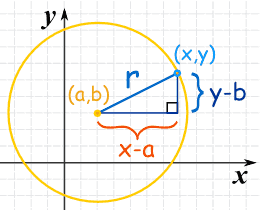# How do you write the standard form of the equation of the circle with the given the center (0,0), r=12?

Apr 19, 2016

${x}^{2} + {y}^{2} = {12}^{2}$

#### Explanation:

For a circle centered at $\left(a , b\right)$ with radius $r$, the equation of the circle is given by

${\left(x - a\right)}^{2} + {\left(y - b\right)}^{2} = {r}^{2}$

This follows from the Pythagoras Theorem. Refer to the diagram below.In this case, $\left(a , b\right) = \left(0 , 0\right)$ and $r = 12$. Therefore, the equation of the circle is

${\left(x - 0\right)}^{2} + {\left(y - 0\right)}^{2} = {12}^{2}$

or after simplifying,

${x}^{2} + {y}^{2} = 144$